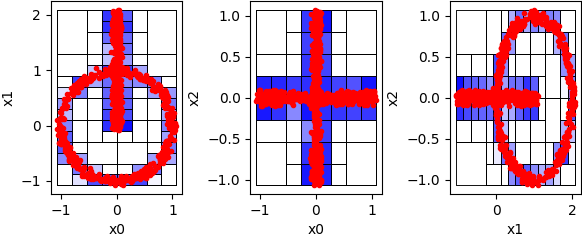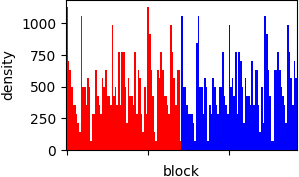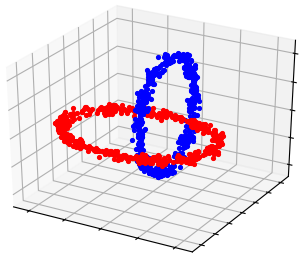pyclustering  0.10.1 pyclustring is a Python, C++ data mining library.
pyclustering.cluster.bang.bang Class Reference

Class implements BANG grid based clustering algorithm. More...

## Public Member Functions

def __init__ (self, data, levels, ccore=False, **kwargs)
Create BANG clustering algorithm. More...

def process (self)
Performs clustering process in line with rules of BANG clustering algorithm. More...

def get_clusters (self)
Returns allocated clusters. More...

def get_noise (self)
Returns allocated noise. More...

def get_directory (self)
Returns grid directory that describes grid of the processed data. More...

def get_dendrogram (self)
Returns dendrogram of clusters. More...

def get_cluster_encoding (self)
Returns clustering result representation type that indicate how clusters are encoded. More...

## Detailed Description

Class implements BANG grid based clustering algorithm.

BANG clustering algorithms uses a multidimensional grid structure to organize the value space surrounding the pattern values. The patterns are grouped into blocks and clustered with respect to the blocks by a topological neighbor search algorithm .

Code example of BANG usage:

from pyclustering.cluster.bang import bang, bang_visualizer
from pyclustering.samples.definitions import FCPS_SAMPLES
# Read data three dimensional data.
# Prepare algorithm's parameters.
levels = 11
# Create instance of BANG algorithm.
bang_instance = bang(data, levels)
bang_instance.process()
# Obtain clustering results.
clusters = bang_instance.get_clusters()
noise = bang_instance.get_noise()
directory = bang_instance.get_directory()
dendrogram = bang_instance.get_dendrogram()
# Visualize BANG clustering results.
bang_visualizer.show_blocks(directory)
bang_visualizer.show_dendrogram(dendrogram)
bang_visualizer.show_clusters(data, clusters, noise)

There is visualization of BANG-clustering of three-dimensional data 'chainlink'. BANG-blocks that were formed during processing are shown on following figure. The darkest color means highest density, blocks that does not cover points are transparent:Fig. 1. BANG-blocks that cover input data.

Here is obtained dendrogram that can be used for further analysis to improve clustering results:Fig. 2. BANG dendrogram where the X-axis contains BANG-blocks, the Y-axis contains density.

BANG clustering result of 'chainlink' data:Fig. 3. BANG clustering result. Data: 'chainlink'.

Definition at line 934 of file bang.py.

## ◆ __init__()

 def pyclustering.cluster.bang.bang.__init__ ( self, data, levels, ccore = False, ** kwargs )

Create BANG clustering algorithm.

Parameters
 [in] data (list): Input data (list of points) that should be clustered. [in] levels (uint): Amount of levels in tree that is used for splitting (how many times block should be split). For example, if amount of levels is two then surface will be divided into two blocks and each obtained block will be divided into blocks also. [in] ccore (bool): Reserved positional argument - not used yet. [in] **kwargs Arbitrary keyword arguments (available arguments: 'observe').

Keyword Args:

• density_threshold (double): If block density is smaller than this value then contained data by this block is considered as a noise and its points as outliers. Block density is defined by amount of points in block divided by block volume: amount_block_points/block_volume. By default it is 0.0 - means than only empty blocks are considered as noise. Be aware that this parameter is used with parameter 'amount_threshold' - the maximum threshold is considered during processing.
• amount_threshold (uint): Amount of points in the block when it contained data in bang-block is considered as a noise and there is no need to split it till the last level. Be aware that this parameter is used with parameter 'density_threshold' - the maximum threshold is considered during processing.

Definition at line 982 of file bang.py.

## ◆ get_cluster_encoding()

 def pyclustering.cluster.bang.bang.get_cluster_encoding ( self )

Returns clustering result representation type that indicate how clusters are encoded.

Returns
(type_encoding) Clustering result representation.
get_clusters()

Definition at line 1094 of file bang.py.

## ◆ get_clusters()

 def pyclustering.cluster.bang.bang.get_clusters ( self )

Returns allocated clusters.

Remarks
Allocated clusters are returned only after data processing (method process()). Otherwise empty list is returned.
Returns
(list) List of allocated clusters, each cluster contains indexes of objects in list of data.
process()
get_noise()

Definition at line 1038 of file bang.py.

## ◆ get_dendrogram()

 def pyclustering.cluster.bang.bang.get_dendrogram ( self )

Returns dendrogram of clusters.

Dendrogram is created in following way: the density indices of all regions are calculated and sorted in decreasing order for each cluster during clustering process.

Remarks
Dendrogram is returned only after data processing (method process()). Otherwise empty list is returned.

Definition at line 1082 of file bang.py.

## ◆ get_directory()

 def pyclustering.cluster.bang.bang.get_directory ( self )

Returns grid directory that describes grid of the processed data.

Remarks
Grid directory is returned only after data processing (method process()). Otherwise None value is returned.
Returns
(bang_directory) BANG directory that describes grid of process data.
process()

Definition at line 1068 of file bang.py.

## ◆ get_noise()

 def pyclustering.cluster.bang.bang.get_noise ( self )

Returns allocated noise.

Remarks
Allocated noise is returned only after data processing (method process()). Otherwise empty list is returned.
Returns
(list) List of indexes that are marked as a noise.
process()
get_clusters()

Definition at line 1053 of file bang.py.

## ◆ process()

 def pyclustering.cluster.bang.bang.process ( self )

Performs clustering process in line with rules of BANG clustering algorithm.

Returns
(bang) Returns itself (BANG instance).
get_clusters()
get_noise()
get_directory()
get_dendrogram()

Definition at line 1018 of file bang.py.

The documentation for this class was generated from the following file:
pyclustering.cluster.bang
Cluster analysis algorithm: BANG.
Definition: bang.py:1
pyclustering.utils
Utils that are used by modules of pyclustering.
Definition: __init__.py:1